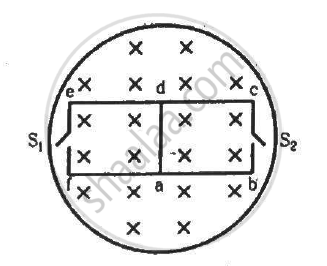Advertisement Remove all ads

# The Magnetic Field in the Cylindrical Region Shown in Figure Increases at a Constant Rate of 20.0 Mt/S. Each Side of the Square Loop Abcd And Defa Has a Length of 1.00 Cm and a Resistance of 4.00 ω. - Physics

Sum

The magnetic field in the cylindrical region shown in figure increases at a constant rate of 20.0 mT/s. Each side of the square loop abcd and defa has a length of 1.00 cm and a resistance of 4.00 Ω. Find the current (magnitude and sense) in the wire ad if (a) the switch S1 is closed but S2 is open, (b) S1 is open but S2 is closed, (c) both S1 and S2 are open and (d) both S1 and S2 are closed.Advertisement Remove all ads

#### Solution

(a) When switch S1 is closed and switch S2 is open:-

Rate of change of the magnetic field = 20 mT/s = $2 \times {10}^{- 4} T/s$

Net resistance of the coil adef, R = 4 × 4 = 16 Ω

Area of the coil adef = (10−2 )−2 = 10−4 m2

The emf induced is given  by

$e = \frac{d\phi}{dt} = A . \frac{dB}{dt}$

= 10−4 × 2 × 10−2

= 2 × 10−6 V

The current through the wire ad is given by

$i= \frac{e}{R} = \frac{2 \times {10}^{- 6}}{16}$

= 1.25 × 10−7 A along ad

(b)  When switch S2 is closed and switch S1 is open:-

Net resistance of the coil abcd, R = 16 Ω

The induced emf is given by

$e = A \times \frac{dB}{dt} = 2 \times {10}^{- 6} V$

The current through wire ad is given by

$i = \frac{20 \times {10}^{- 6}}{16} = 1 . 25 \times {10}^{- 7} A$ along da

(c) When both S1 and S2 are open, no current is passed, as the circuit is open. Thus, i = 0.

(d) When both S1 and S2 are closed, the circuit forms a balanced a Wheatstone bridge and no current flows along ad. Thus, i = 0.

Concept: Induced Emf and Current
Is there an error in this question or solution?
Advertisement Remove all ads

#### APPEARS IN

HC Verma Class 11, Class 12 Concepts of Physics Vol. 2
Chapter 16 Electromagnetic Induction
Q 19 | Page 307
Advertisement Remove all ads

#### Video TutorialsVIEW ALL 

Advertisement Remove all ads
Share
Notifications

View all notifications

Forgot password?